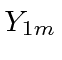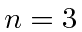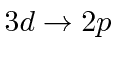## Homework Problems

1. The interaction term for Electric Quadrupole transitions correspond to a linear combination of spherical harmonics,, and are parity even. Find the selection rules for E2 transitions.

2. Magnetic dipole transitions are due to an axial vector operator and hence are proportional to thebut do not change parity (unlike a vector operator). What are the M1 selection rules?

3. Draw the energy level diagram for hydrogen up to. Show the allowed E1 transitions. Use another color to show the allowed E2 and M1 transitions.

4. Calculate the decay rate for thetransition.

5. Calculate the decay rate for thetransition in hydrogen.

6. Assume that we prepare Hydrogen atoms in thestate. We set up an experiment with the atoms at the origin and detectors sensitive to the polariztion along each of the 3 coordinate axes. What is the probability that a photon with its wave vector pointing along the axis will be Left Circularly Polarized?

7. Photons from thetransition are observed coming from the sun. Quantitatively compare the natural line width to the widths from Doppler broadening and collision broadening expected for radiation from the sun's surface.

Jim Branson 2013-04-22